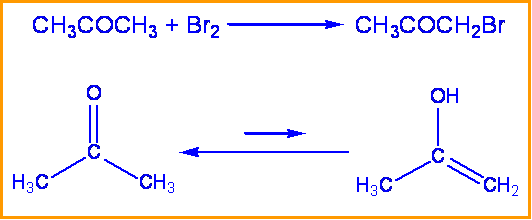# 6.17: The E2 Reaction and the Deuterium Isotope Effect

$$\newcommand{\vecs}{\overset { \rightharpoonup} {\mathbf{#1}} }$$ $$\newcommand{\vecd}{\overset{-\!-\!\rightharpoonup}{\vphantom{a}\smash {#1}}}$$$$\newcommand{\id}{\mathrm{id}}$$ $$\newcommand{\Span}{\mathrm{span}}$$ $$\newcommand{\kernel}{\mathrm{null}\,}$$ $$\newcommand{\range}{\mathrm{range}\,}$$ $$\newcommand{\RealPart}{\mathrm{Re}}$$ $$\newcommand{\ImaginaryPart}{\mathrm{Im}}$$ $$\newcommand{\Argument}{\mathrm{Arg}}$$ $$\newcommand{\norm}{\| #1 \|}$$ $$\newcommand{\inner}{\langle #1, #2 \rangle}$$ $$\newcommand{\Span}{\mathrm{span}}$$ $$\newcommand{\id}{\mathrm{id}}$$ $$\newcommand{\Span}{\mathrm{span}}$$ $$\newcommand{\kernel}{\mathrm{null}\,}$$ $$\newcommand{\range}{\mathrm{range}\,}$$ $$\newcommand{\RealPart}{\mathrm{Re}}$$ $$\newcommand{\ImaginaryPart}{\mathrm{Im}}$$ $$\newcommand{\Argument}{\mathrm{Arg}}$$ $$\newcommand{\norm}{\| #1 \|}$$ $$\newcommand{\inner}{\langle #1, #2 \rangle}$$ $$\newcommand{\Span}{\mathrm{span}}$$$$\newcommand{\AA}{\unicode[.8,0]{x212B}}$$

Learning Objective

• explain how the kinetic isotope effect (KIE) can be used to elucidate reaction mechanisms

## Kinetic Isotope Effects

Kinetic Isotope Effects (KIEs) are used to determine reaction mechanisms by determining rate limiting steps and transition states and are commonly measured using NMR to detect isotope location or GC/MS to detect mass changes. In a KIE experiment an atom is replaced by its isotope and the change in rate of the reaction is observed. A very common isotope substitution is when hydrogen is replaced by deuterium. This is known as a deuterium effect and is expressed by the ratio kH/kD (as explained above). Normal KIEs for the deuterium effect are around 1 to 7 or 8. Large effects are seen because the percentage mass change between hydrogen and deuterium is great. Heavy atom isotope effects involve the substitution of carbon, oxygen, nitrogen, sulfur, and bromine, with effects that are much smaller and are usually between 1.02 and 1.10. The difference in KIE magnitude is directly related to the percentage change in mass. Large effects are seen when hydrogen is replaced with deuterium because the percentage mass change is very large (mass is being doubled) while smaller percent mass changes are present when an atom like sulfur is replaced with its isotope (increased by two mass units).

### Primary KIEs

Primary kinetic isotope effects are rate changes due to isotopic substitution at a site of bond breaking in the rate determining step of a reaction.

Example

Consider the bromination of acetone: kinetic studies have been performed that show the rate of this reaction is independent of the concentration of bromine. To determine the rate determining step and mechanism of this reaction the substitution of a deuterium for a hydrogen can be made.When hydrogen was replaced with deuterium in this reaction a $$k_H \over k_D$$ of 7 was found. Therefore the rate determining step is the tautomerization of acetone and involves the breaking of a C-H bond. Since the breaking of a C-H bond is involved, a substantial isotope effect is expected.